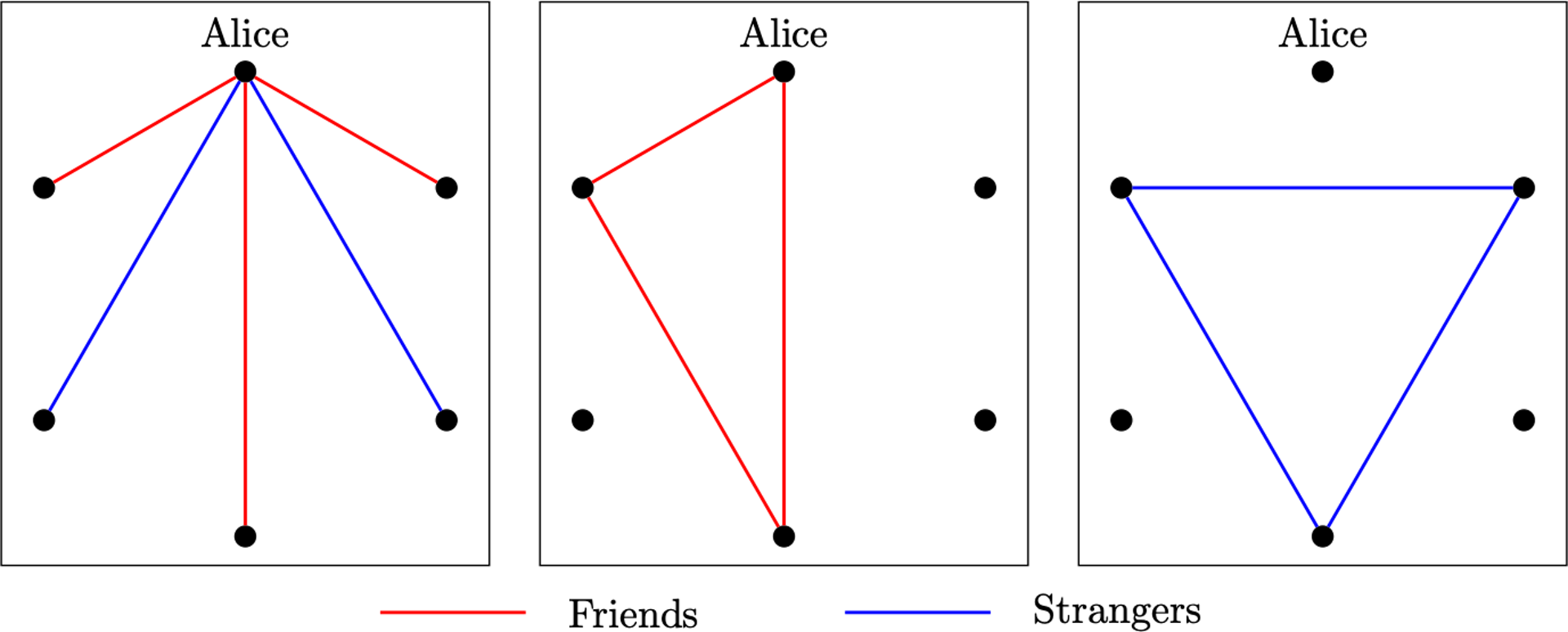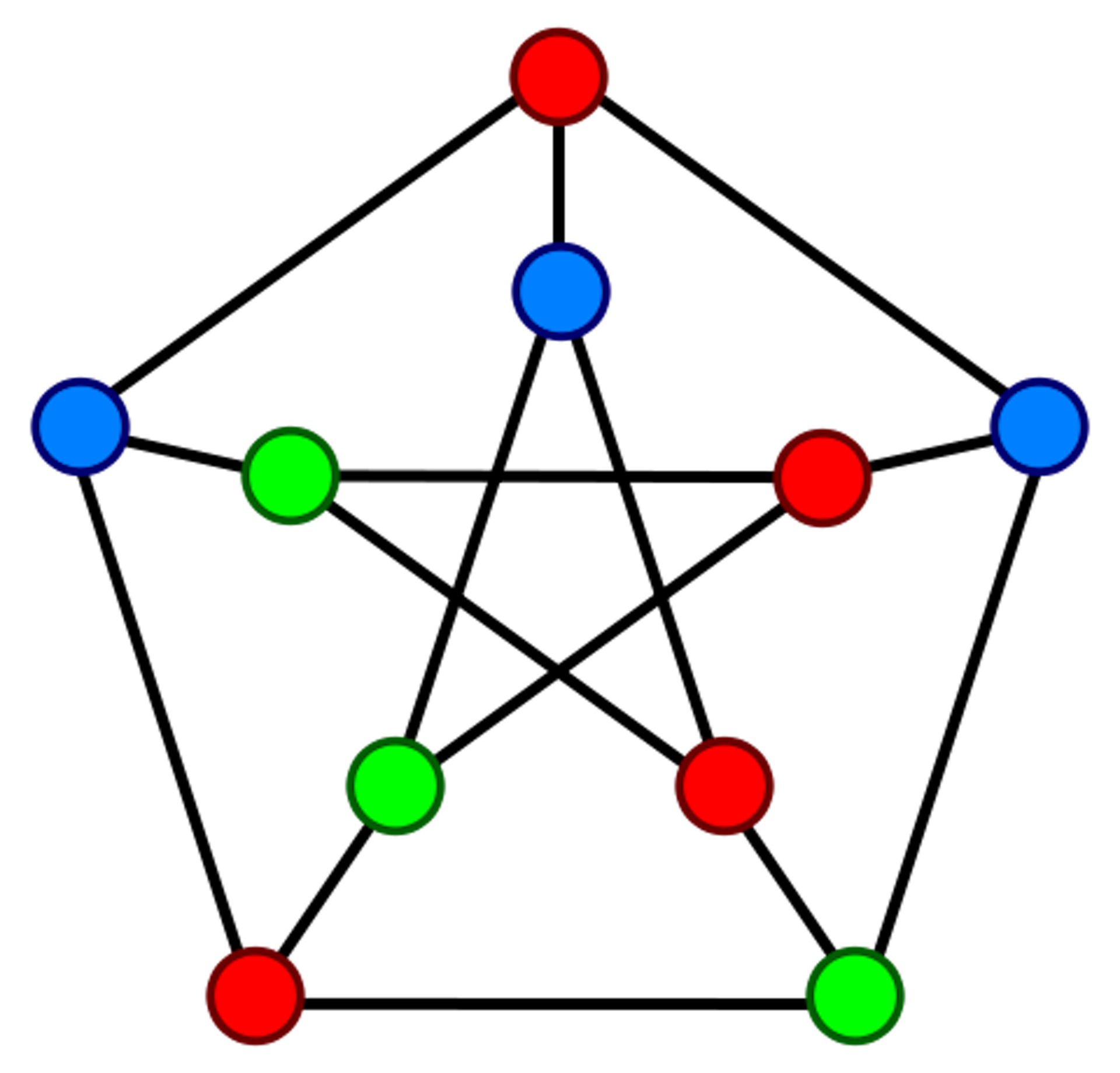# Why Facebook belongs in the math classroom

Some of your friends are also friends with each other, while others are not. It’s quite likely that you can find a “clique” who are all friends with each other.

It’s also possible you may have a group of Facebook friends who are all mutual strangers, where nobody is friends with anybody else in the group; let’s call such a group an “anti-clique.”

It wouldn’t take long to find a clique or anti-clique of at least three people. In fact, among any six people, there will be a group of three that forms either a clique or an anti-clique.

This exercise might seem frivolous, but there’s an underlying structure here that serves as an effective illustration of certain advanced mathematics. I use examples like these in the classroom to engage undergraduates in mathematical reasoning without detailed computations.

## Graphing cliques

Let’s return to the problem of finding a clique or anti-clique. Consider a girl called Alice who is spending time with five people. Among these five, Alice must see either more friends or more strangers.

If Alice sees more friends than strangers, that means there are at least three people who are all friends with Alice. If any pair within these three people are friends, then they form a clique of three people with Alice. If none of these three are friends, then we have found an anti-clique of three people.Left: Alice sees three friends and two strangers; Center: If any pair of Alice’s friends are also friends, then they form a clique with Alice; Right: If none of Alice’s friends knows each other, the three form an anti-clique. Jonathan Hulgan, CC BY-SA

The same situation would work if you assumed Alice saw more strangers than friends.

This argument is a relatively simple result from the area of pure mathematics known as graph theory.

In mathematics, graphs sometimes refer to the graphical representation of functions, such as lines, parabolas and other curves. Graph theory studies something different. It focuses on the properties of mathematical structures that abstractly model relationships between objects. The objects can be represented by points, called vertices; related objects would have their corresponding vertices connected by a line called an edge.

In the Facebook example, a graph theorist would consider a graph whose vertices represent the collection of friends. An edge would connect each pair of individuals who were Facebook friends with each other.

Precisely speaking, when a graph is defined by a relation on some collection of objects, as is the case here, it is called a “network.” So Facebook truly describes a “social network.”A Petersen graph, a common example used in graph theory problems. Wikimedia

Most students I encounter have never studied graph theory; it’s rare for it to appear in high school or a lower-level math course.

This is unfortunate, as I feel that graph theory provides a perfect setting for practicing mathematical arguments without requiring tedious calculations – just as in the example with Alice and her Facebook friends.

What’s more, graph theory lends itself nicely to the development of questions of real mathematical substance. My simple demonstration of the presence of a three-person clique or anti-clique in any group of six people can motivate further questions from the curious observer: When can you guarantee a four-person clique or anti-clique? If three relationships are possible between individuals – for instance, friends, strangers and acquaintances – when would you be guaranteed to see a three-person clique, anti-clique or “pseudo-clique” of mere acquaintances?

These and similar questions were answered in 1955 by mathematicians Robert Greenwood and Andrew Gleason. The mathematical contributions of Greenwood and Gleason are profound and numerous. However, no mathematical expertise is required to develop such questions, nor is it needed to enjoy the pursuit of a complete solution.

## Teaching graph theory

This August, I will teach a course entitled “Graph Theory: Problems, Proofs and Conjectures,” specifically geared toward incoming first-year students. Using concepts from graph theory, my course will introduce students to the process by which knowledge is developed and discovered in mathematics.

Many math courses focus on developing the tools for demonstrating the logical truth of mathematical statements. While my seminar will include a healthy dose of this – including fundamentals of writing formal mathematical proof – it will also require students to develop novel mathematical statements for which it is unknown whether its outcome is true or false.

One of the first problems we will examine is the Facebook friends problem described earlier. I will not bring up Greenwood and Gleason’s work, but I expect at least one or two students will pose some variation of the problems they considered.

Just as graph theory is one area among many within the larger field of mathematics, the types of problems presented here represent only one sort of question considered by graph theorists. My seminar will investigate questions from other parts of graph theory, including the famous traveling salesman problem – for which there exist a number of curious applications – and graph coloring problems, which are my particular interest.

In my view, graph theory illuminates an underlying nature of mathematics that can often seem hidden behind the numbers and computations of algebra and calculus. Introducing questions such as these in the classroom can let students see a side of mathematics they might not have considered before.